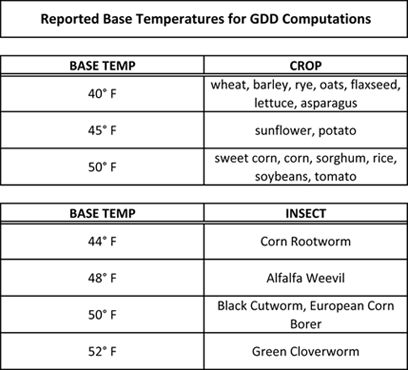## Growing Degree Days:

Growing Degree Days (GDD) are used to estimate the growth and development of plants and insects during the growing season. The basic concept is that development will only occur if the temperature exceeds some minimum development threshold, or base temperature (TBASE). The base temperatures are determined experimentally and are different for each organism.To calculate GDDs, you must first find the mean temperature for the day. The mean temperature is found by adding together the high and low temperature for the day and dividing by two. If the mean temperature is at or below TBASE, then the Growing Degree Day value is zero. If the mean temperature is above TBASE, then the Growing Degree Day amount equals the mean temperature minus TBASE. For example, if the mean temperature was 75° F, then the GDD amount equals 10 for a TBASE of 65° F. You can think of Growing Degree Days as similar to Cooling Degree Days, only the base temperature can be something besides 65° F.

In equation form:Where:
TBASE = Growing Degree Day base temperature
TMEAN = mean temperature, (TMAX + TMIN) / 2

## Modified Growing Degree Days:

Modified Growing Degree Days are similar to Growing Degree Days with several temperature adjustments. If the high temperature is above 86° F, it is reset to 86° F. If the low is below 50° F, it is reset to 50° F. Once the high / low temperatures have been modified (if needed), the average temperature for the day is computed and compared with a base temperature, which is usually 50° F. Modified Growing Degree Days are typically used to monitor the development of corn, the assumption being that development is limited once the temperature exceeds 86° F or falls below 50° F. For example, if the high for the day was 92° F and the low 68° F, the average for use in the modified GDD calculation would be 86 + 68 = 154 / 2 = 77.